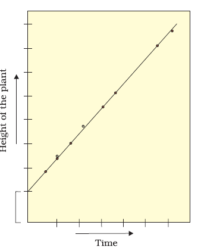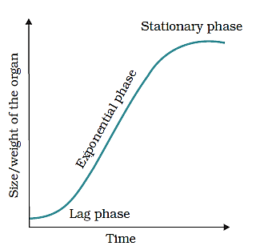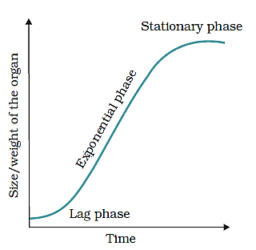# Chapter 15 – Plant Growth and Development Questions and Answers: NCERT Solutions for Class 11 Biology

Class 11 Biology NCERT book solutions for Chapter 15 - Plant Growth and Development Questions and Answers.## 3. Describe briefly: (a) Arithmetic growth (b) Geometric growth (c) Sigmoid growth curve (d) Absolute and relative growth rates

### Solution: (a)In arithmetic growth, only one daughter cell continues to divide while the other differentiates and matures. The simplest expression of arithmetic growth is exemplified by a root elongating at a constant rate.### It can be mathematically expressed as follows: Lt= LO+ rt Lt= length of time ‘t’ LO= length at time ‘zero’ r= growth rate/elongation per unit time (b)In geometric growth, the initial growth is slow (lag phase), and it increases rapidly after that – at an exponential rate (log or exponential phase). Here, both the progeny cells following mitotic cell division retain the ability to divide and continue to do so. The growth slows down due to limited nutrient supply leading to stationary phase. The number increases in a multiplicative pattern in geometric growth.### In geometric growth if we plot the parameter of growth against time, we get a typical sigmoid or S-curve. Exponential growth can be expressed as: W1= W0ert W1= final size W0= initial size of the period r=growth rate t=time growth e=base of natural logarithms ‘r’ is the relative growth as well as the measure of the ability of plants to generate new plant substances which is termed as efficiency index. Thus, the final size of W1is dependant on the initial size W0 (c) Sigmoid growth curve It is an S – shaped graph that is generated by plotting growth against time and has four main components – a slow lag phase, exponential phase or rapid phase, stage of diminishing growth and stationary phase.